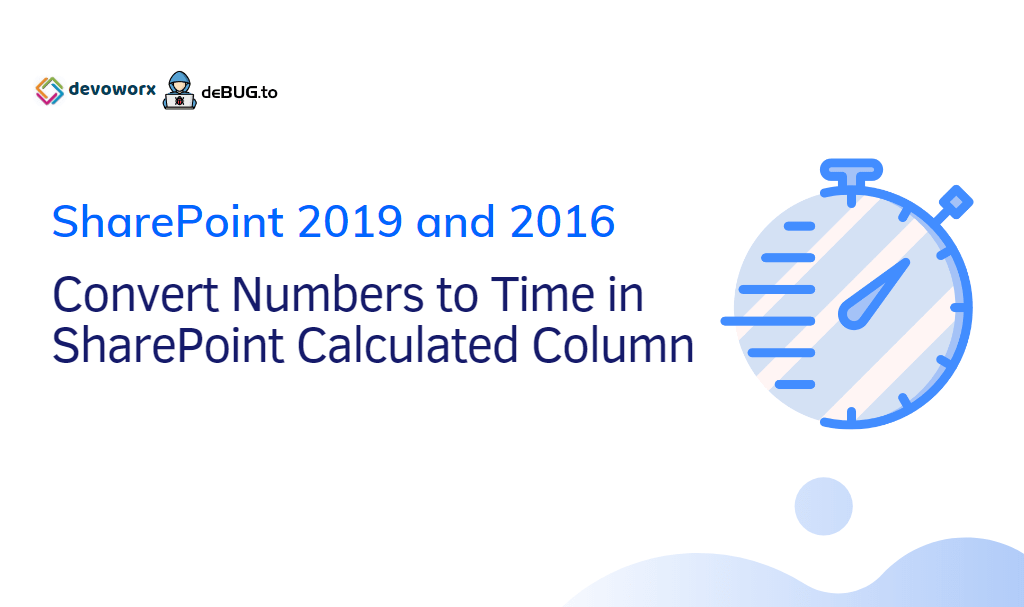# Convert Number To Time in SharePoint Calculated FieldIn this post, we will explain How to convert Number to time in SharePoint Calculated Field

You might also like to read Supported and Unsupported Columns In SharePoint Calculated Column Formula

## Convert Number to Duration SharePoint Calculated Field

I have a SharePoint list, one of its columns is a number column that holds a duration value with minutes.

I would like to display the number of minutes as a Time Format (hh:mm) in the SharePoint Calculated field as shown below:

## Convert Number To Time in SharePoint Calculated Field

in this section, we’ll show how to display a decimal as a time in SharePoint calculated column by doing the below steps:

1. Create a SharePoint List.
2. Add a new number column for the minute’s value.
3. Create a calculated field to convert the minute’s value to time format (hh:mm).

### Create a new SharePoint List

#### Steps

• Open SharePoint site.
• Click on the setting gear icon, click on “Add a new App”.
• Create a custom list.

### Create a Number Column in SharePoint List

In the newly created SharePoint List, we’ll create a new column for “Minutes” with Number datatype by doing the following:

#### Steps

• Open List Settings.
• Scroll down to columns, click on add a new column.
• The field data type must be a Number.
• The number of decimal places should be 0.

### Create a Time Column in SharePoint List

• Set the data type returned from this formula to Number with 0 number of decimal places.
• Save the formula.

### Convert Minutes to Time Format in SharePoint Calculated Column

• The newly calculated field should calculate the time as an hour and minutes based on the assigned number in the minute’s column.

Also, you can use any online converter tool to make sure that the calculation has been done successfully.

### Time Format In SharePoint Calculated Field

In this section, we’ll explain in details the formula used to Convert Number To Time in SharePoint Calculated Field.

#### Hour Calculation

we have calculated the hours by dividing the number of minutes by 60 (Minutes / 60) then we used INT function to round down the result to the nearest integer.

#### Minute Calculation

we have also calculated the Minutes by getting the fraction result of (Minutes / 60) then multiply this fraction to 60.

Note: Use ROUND(number,num_digits) function to round a specific number to a specified number of digits, when the number of digits is equal to zero so it round to the nearest integer as I did in my formula.

##### Conclusion

In conclusion, we have learned How to Convert Number To Time in SharePoint Calculated Field, also we have explored the use of

• INT function in the SharePoint calculated field.
• ROUND function in the SharePoint calculated field.

You can download the list template with the time formula from GitHub at Convert Minutes to Time format in SharePoint Calculated Field, also Please, don’t forget to Follow Me to get the latest updates.

###### Applies To
• SharePoint Online.
• SharePoint 2019.
• SharePoint 2016.
• SharePoint 2013.
• SharePoint 2010.
###### Have a Question?

If you have any related questions, please don’t hesitate to ask it at deBUG.to Community.

### 8 thoughts on “Convert Number To Time in SharePoint Calculated Field”

1.This is pretty helpful, thank you. How would the formula be written to convert the value of a “Seconds” column in hh:mm:ss format? I just don’t know where to put the “/3600”.

Scroll to Top